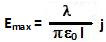## Tuesday, May 4, 2010

### Irodov Problem 3.22First let us determine the electric field due to an infinitely long thread at any point in space. The easiest way to do this is using Gauss law which says,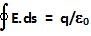Here, E is the electric field vector at a point on the Gaussian surface, dS is an infinitesimally small area vector pointing in a direction normal to the Gaussian surface. q is the totial charge contained within the Gaussian surface. In this problem, since the thread is infinitely long, at any point in space the electric field will point radially outwards as shown in Fig1. So, if we choose a Gaussian surface that is in the shape of a cylinder or radius r with its axis coinciding with that of the thread, then at each point on the cylinder's surface, the directions of E and dS will coincide and further, the magnitude of the electric field E will also be the same. Suppose that the length of cylinder is L, then, this means that,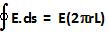The total charge contained in the cylinder will be,Hence, from Gauss Law we can write,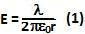and E will be directed radially outwards from the cylinder as shown in Figure 1.The situation of the problem is indicated in the Figure above. The question essentially asks at what distance x will the net electric field due to the two charged threads be maximum. Suppose that the point at a distance x is at an angle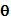with the line connecting the charges i.e.Whenis close to 0, the electric fields from both the threads simply cancel each other, whenbecomes to large then the distance of the point from the threads is so large that the magnitude of the electric field strength itself becomes small. So there must be an optimal angle at which electric field strength becomes maximum.

As seen in the top view (the threads are coming out of the screen towards your face), based on elementary trigonometry, the distance of the point from each of the threads is given by,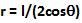Hence, the electric fields due to the left and right threads are given by,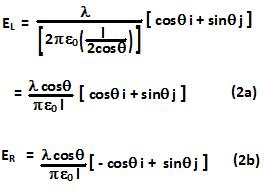The net electric field is thus given by,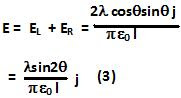From (3) the magnitude of the electric field vector is maximum whenand the electric field strength is,